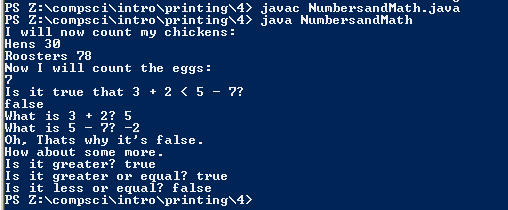# Assignemnt #8

## Code

```  /// Name: Sam Wilson
/// Period: 6
/// Program Name: First Program
/// File Name: FirstProg.java
/// Date Finished: 9/14/2015

public class NumbersandMath
{
public static void main( String[] args)
{

System.out.println("I will now count my chickens:");
System.out.println("Hens " + ( 25 + 30 / 6 ));
//subtracting and the % gives you the remainder
System.out.println("Roosters " + ( 100-25 +3%4));

System.out.println("Now I will count the eggs:");
//adding subtracting dividing and the remainder
System.out.println( 3+2+1-5+4%2-1/4+6);

System.out.println("Is it true that 3 + 2 < 5 - 7? ");
// adding subtracting and < less than
System.out.println( 3+2<5-7);
//The numbers are in a string than in a () causing it to be read as an equation
System.out.println("What is 3 + 2? " + ( 3+2));
System.out.println("What is 5 - 7? " + ( 5-7));

System.out.println("Oh, Thats why it's false.");